Squares in Triangles

The following pictures show n unit squares packed inside the smallest known equilateral triangles (of side length s).

 1.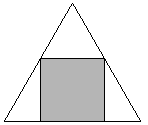2.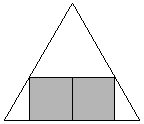3.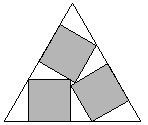s = 1 + 2 / √3 = 2.154+Trivial. s = 2 + 2 / √3 = 3.154+Trivial. s = 3 / 2 + √3 = 3.232+Found by Erich Friedmanin 1997.

 4.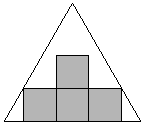5.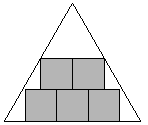6.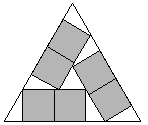s = 3 + 2 / √3 = 4.154+Found by Erich Friedmanin 1997. s = 2 + 4 / √3 = 4.309+Found by Erich Friedmanin 1997. s = 2 + 4/ √3 = 4.309+Found by Erich Friedmanin 1997.

 7.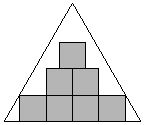8.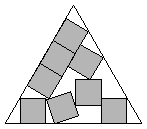9.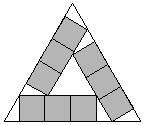s = 4 + 2 / √3 = 5.154+Found by Erich Friedmanin 1997. s = 5.301+Found by David W. Cantrellin July 2002. s = 3 + 4 / √3 = 5.309+Found by Erich Friedmanin 1997.

 10.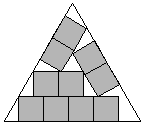11.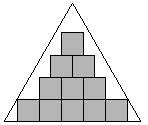12.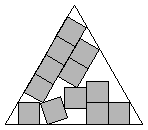s = 2 + 2 √3 = 5.464+Found by Erich Friedmanin 1997. s = 5 + 2 / √3 = 6.154+Found by Erich Friedmanin 1997. s = 6.301+Found by David W. Cantrellin July 2002.

 13.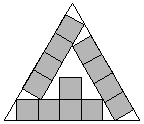14.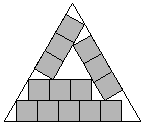15.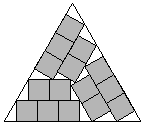s = 4 + 4 / √3 = 6.309+Found by Erich Friedmanin 1997. s = 3 + 2 √3 = 6.464+Found by Erich Friedmanin 1997. s = 3 + 2 √3 = 6.464+Found by Erich Friedmanin 1997.

 16.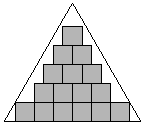17.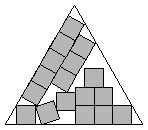18.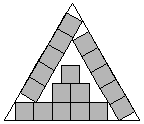s = 6 + 2 / √3 = 7.154+Found by Erich Friedmanin 1997. s = 7.301+Found by David W. Cantrellin July 2002. s = 5 + 4 / √3 = 7.309+Found by Erich Friedmanin 1997.

 19.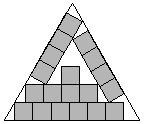20.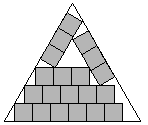21.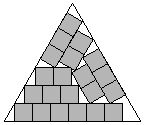s = 4 + 2 √3 = 7.464+Found by Erich Friedmanin 1997. s = 3 + 8 / √3 = 7.618+Found by Erich Friedmanin 1997. s = 3 + 8 / √3 = 7.618+Found by Erich Friedmanin 1997.

 22.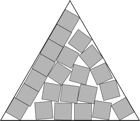23.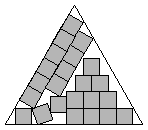24.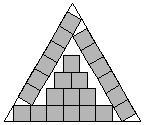s = 7 + 2 / √3 = 8.154+Found by Erich Friedmanin 1997. s = 8.301+Found by David W. Cantrellin July 2002. s = 6 + 4 / √3 = 8.309+Found by Erich Friedmanin 1997.

 25.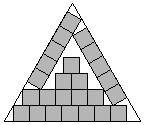26.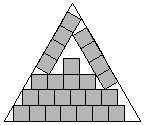27.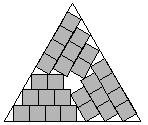s = 5 + 2 √3 = 8.464+Found by Erich Friedmanin 1997. s = 4 + 8 / √3 = 8.618+Found by Erich Friedmanin 1997. s = 4 + 8 / √3 = 8.618+Found by Erich Friedmanin 1997.

 28.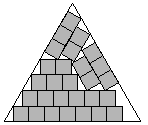29.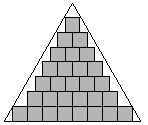30.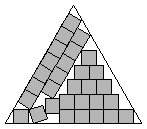s = 3 + 10 / √3 = 8.773+Found by Erich Friedmanin 1997. s = 8 + 2 / √3 = 9.154+Found by Erich Friedmanin 1997. s = 9.301+Found by David W. Cantrellin July 2002.

 31.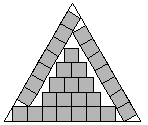32.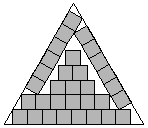33.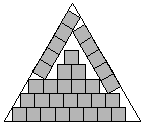s = 7 + 4 / √3 = 9.309+Found by Erich Friedmanin 1997. s = 6 + 2 √3 = 9.464+Found by Erich Friedmanin 1997. s = 5 + 8 / √3 = 9.618+Found by Erich Friedmanin 1997.

 34.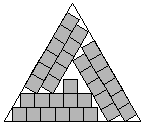35.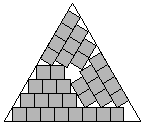36.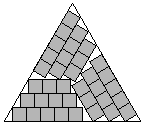s = 5 + 8 / √3 = 9.618+Found by Erich Friedmanin 1997. s = 4 + 10 / √3 = 9.773+Found by Erich Friedmanin 1997. s = 4 + 10 / √3 = 9.773+Found by Erich Friedmanin 1997.